## How to Calculate and Solve for the Width, Height, Length and Volume of a Rectangular Prism | Nickzom Calculator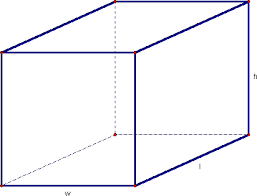The image above is a rectangular prism.

To compute the volume of a rectangular prism requires three essential parameters which are the length, width and height of the rectangular prism.

V = lwh

Where;
V = Volume of the rectangular prism
l = Length of the rectangular prism
w = Width of the rectangular prism
h = Height of the rectangular prism

Let’s solve an example;
Find the volume of a rectangular prism with a length of 18 cm, a width of 12 cm and a height of 21 cm.

This implies that;
l = Length of the rectangular prism = 18 cm
w = Width of the rectangular prism = 12 cm
h = Height of the rectangular prism = 21 cm

V = lwh
V = 18 x 12 x 21
V = 4536

Therefore, the volume of the rectangular prism is 4536 cm3.

Calculating the Length of a Rectangular Prism using the Volume, Width and Height of the Rectangular Prism.

l = V / wh

Where;
l = Length of the rectangular prism
V = Volume of the rectangular prism
w = Width of the rectangular prism
h = Height of the rectangular prism

Let’s solve an example;
Find the length of a rectangular prism with a width of 19 cm, a height of 24 cm and a Volume of 250 cm3.

This implies that;
w = Width of the rectangular prism = 19 cm
h = Height of the rectangular prism = 24 cm
V = Volume of the rectangular prism = 250 cm3

l = V / wh
l = 250 / 19 x 24
l = 250 / 456
l = 0.55

Therefore, the length of the rectangular prism is 0.55 cm.

## How to Calculate and Solve for the Length of a Side and Perimeter of a Cube | The Calculator Encyclopedia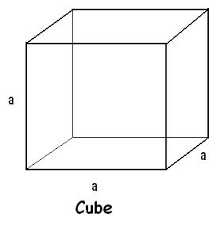The image above is a cube.

To compute the perimeter of a cube, one essential parameter is needed and this parameter is length of one of the sides (a).

The formula for calculating the perimeter of a cube:

P = 12a

Where;
P = Perimeter of the cube
a = Length of one of the sides of the cube

Let’s solve an example;
Find the perimeter of a cube when the length of one of the sides is 14 cm.

This implies that;
a = Length of one of the sides = 14 cm

P = 12a
P = 12 x 14
P = 168

Therefore, the perimeter of the cube is 168 cm.

Calculating the Length of one of the sides of a Cube using the Perimeter of the Cube.

a = P / 12

Where;
a = Length of one of the sides of the cube
P = Perimeter of the cube

Let’s solve an example;
Find the length of one of the sides of a cube when the perimeter of the cube is 200 cm.

This implies that;
P = Perimeter of the cube = 200 cm

a = P / 12
a = 200 / 12
a = 16.67

Therefore, the length of one of the sides is 16.67 cm.

## How to Calculate and Solve for the Perimeter of an Ellipse | Nickzom Calculator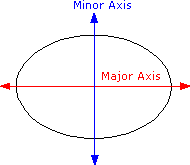The image above is an ellipse.

To compute the perimeter of an ellipse, two essential parameters are needed and this parameters are axis (a) and axis (b).

The formula for calculating the perimeter of an ellipse:

P = π [3(a + b) – √((3a + b)(a + 3b))]

Where;

P = Perimeter of the ellipse
a = Axis of the ellipse
b = Axis of the ellipse

Let’s solve an example;
Given that the axis of the ellipse (a) is 19 cm and axis of the ellipse (b) is 31 cm. Find the perimeter of the ellipse?

This implies that;
a = Axis of the ellipse = 19 cm
b = Axis of the ellipse = 31 cm

P = π [3(a + b) – √((3a + b)(a + 3b))]
P = 3.142 [3(19 + 31) – √((3 x 19 + 31)(19 + 3 x 31))]
P = 3.142 [3(50) – √((57+ 31)(19 + 93))]
P = 3.142 [150 – √((88)(112))]
P = 3.142 [150 – √(9856)]
P = 3.142 [150 – 99.28]
P = 3.142 [50.72]
P = 3.142 x 50.72
P = 159.26

Therefore, the perimeter of the ellipse is 159.26 cm.

## How to Calculate and Solve for the Height, Base Edge and Volume of a Square Pyramid | The Calculator Encyclopedia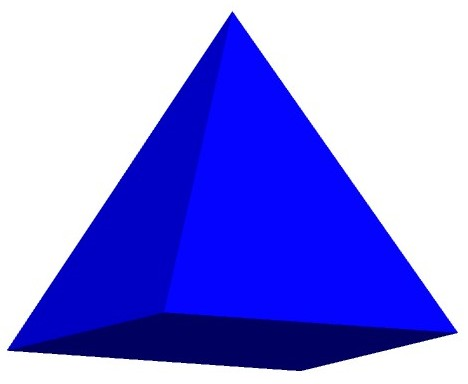The image above represents a square pyramid.
To compute the volume of a square pyramid requires two essential parameters which are the base edge and height of the square pyramid.

The formula for computing the volume of a square pyramid is:

V = ha² / 3

Where:
V = Volume of the Square Pyramid
a = Base edge of the Square Pyramid
h = Height of the Square Pyramid

Let’s solve an example
Find the volume of a square pyramid with a base edge of 6 cm and a height of 11 cm.

This implies that:
a = base edge of the square pyramid = 6
h= = height of the square pyramid = 11

V = ha² / 3
V = 11(6)² / 3
V = 11(36) / 3
V = 396 / 3
V = 132

Therefore, the volume of the square pyramid is 132 cm3.

Calculating the Base edge of a square pyramid when Volume and Height are Given

The formula is a = √(3V / h)

Where;
V = Volume of the Square Pyramid
a = Base edge of the Square Pyramid
h = Height of the Square Pyramid

Let’s solve an example:
Find the base edge of a square pyramid with a volume of 50 cm3 and a height of 20 cm.

This implies that;
V = Volume of the square pyramid = 50 cm3
h  = height of the square pyramid = 20 cm

a = √(3V / h)
a = √(3(50) / 20)
a = √(150 / 20)
a = √7.5
a = 2.739

Therefore, the base edge of the square pyramid is 2.739 cm.

Calculating the height of a square pyramid when Volume and Base edge are Given

The formula is h = 3V / a2

Where;
a = Base edge of the square pyramid
V = Volume of the Square Pyramid
h = Height of the Square Pyramid

Let’s solve an example:
Find the height of a square pyramid with a volume of  250 cm3  and a base edge of 7 cm2

This implies that;
V = Volume of the square pyramid = 250 cm3
a = Base edge of the square pyramid = 7 cm

h =  3V /
h = 3(250) /
h = 750 / 49
h = 15.306

Therefore, the height of the square pyramid is 15.306 cm.

## How to Calculate and Solve for the Axis and Area of an Ellipse | Nickzom Calculator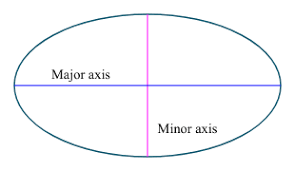The image above is an ellipse.

To compute the area of an ellipse, two essential parameters are needed and this parameters are axis (a) and axis (b).

A = πab

Where;
A = Area of the ellipse
a = Axis of the ellipse
b = Axis of the ellipse

Let’s solve an example;
Find the area of an ellipse when it has an axis (a) of 10 cm and an axis (b) of 17 cm.

This implies that;
a = Axis of the ellipse = 10 cm
b = Axis of the ellipse = 17 cm

A = πab
A = 3.142 x 10 x 17
A = 534.14

Therefore, the area of the ellipse is 534.14 cm2.

Calculating the Axis (a) of an ellipse using Area of the ellipse and Axis (b) of the ellipse.

a = A / πb

Where;
a = Axis of the ellipse
A = Area of the ellipse
b = Axis of the ellipse

Let’s solve an example;
Find the axis (a) of an ellipse when the area of the ellipse is 210 cm2 with an axis (b) of 19 cm.

This implies that;
A = Area of the ellipse = 210 cm2
b = Axis of the ellipse = 19 cm

a = A / πb
a = 210 / 3.142 x 19
a = 210 / 59.70
a = 3.52

Therefore, the axis (a) of an ellipse is 3.52 cm.

## How to Calculate the Volume, Base Area and Height of a Prism | The Calculator EncyclopediaThe image above represents a prism.
To compute the volume of a prism requires two essential parameters which are the base area and height of the prism.

The formula for computing the volume of a prism is:

V = Ah

Where:
V = Volume of the Prism
A = Base area of the Prism
h = Height of the prism

Let’s solve an example
Find the volume of a prism with a base area of 30 cm2 and a height of 8 cm.

This implies that:
A = base area of the prism = 30
h= = height of the prism = 8

V = Ah
V = 30 x 8
V = 240

Therefore, the volume of the prism is 240 cm3.

Calculating the Base area of a prism when Volume and Height are Given

The formula is A = V / h

Where;
V = Volume of the Prism
A = Base area of the Prism
h = Height of the Prism

Let’s solve an example:
Find the base area of a prism with a volume of 300 cm3 and a height of 12 cm.

This implies that;
V = Volume of the prism = 300 cm3
h  = height of the prism = 12 cm

A =  V / h
A = 300 / 12
A = 25

Therefore, the base area of the prism is 25 cm2.

Calculating the height of a prism when Volume and Base area are Given

The formula is h = V / A

Where;
A = Base area of the Prism
V = Volume of the Prism
h = Height of the Prism

Let’s solve an example:
Find the height of a prism with a volume of  280 cm3  and a base area of 35 cm2

This implies that;
V = Volume of the prism = 280 cm3
A = Base area of the prism = 35 cm2

h =  V / A
h = 280 / 35
h = 8

Therefore, the height of the prism is 8 cm.

## How to Calculate and Solve for the Angle, Radius and Length of an Arc of a Circle | The Calculator Encyclopedia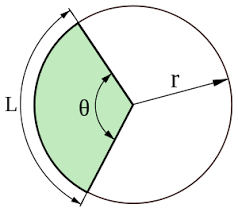The image above represents the length of an arc of a circle.

To compute the length of an arc of a circle, two essential parameters are needed and this parameters are radius of the circle (r) and angle of the circle (α).

The formula for calculating the length of an arc of a circle:

L = απr / 180

Where;
L = Length of an arc of the circle
α = Angle of the circle
r = radius of the circle

Let’s solve an example:
Find the length of an arc of a circle when the angle of the circle is 90° and the radius of the circle is 20 cm.

This implies that;
α = Angle of the circle = 90°
r = Radius of the circle = 20 cm

L = απr / 180
L = 90 x 3.142 x 20 / 180
L = 5655.6 / 180
L = 31.42

Therefore, the length of an arc of the circle is 31.42 cm.

Calculating the Angle of a Circle using the Radius of the Circle and Length of an Arc of the Circle.

α = 180L / πr

Where;
L = Length of an arc of the circle
r = Radius of the circle

Let’s solve an example;
Find the angle of the circle when the length of an arc of the circle is 60° and a radius of 140 cm.

This implies that;
L = Length of an arc of the circle = 60°
r = Radius of the circle = 140 cm

α = 180L / πr
α = 180 x 60 / 3.142 x 140
α = 10800 / 439.88
a = 24.55

Therefore, the angle of the circle is 24.55°.

## How to Calculate and Solve for the Length of a Side and Perimeter of a Hexagon | The Calculator EncyclopediaThe image above is a hexagon.

To compute the perimeter of a hexagon, one essential parameter is needed and this parameter is length of side (a).

The formula for calculating the perimeter of a pentagon:

P = 6a

Where;
P = Perimeter of the hexagon
a = length of side

Let’s solve an example;
Find the perimeter of a hexagon when the length of side is 15 cm.

This implies that;
a = length of side = 15 cm

P = 6a
P = 6 x 15
P = 90

Therefore, the perimeter of the hexagon is 90 cm.

Calculating the length of side (a) using the Perimeter of the hexagon.

a = P / 6

Where;
a = length of side
P = Perimeter of the hexagon

Let’s solve an example;
Find the length of side when the perimeter of the hexagon is 120 cm.

This implies that;
P = Perimeter of the hexagon = 120 cm

a = P / 6
a = 120 / 6
a = 20

Therefore, the length of side (a) is 20 cm.

## How to Calculate and Solve for the Length of a Side and Area of a Hexagon | The Calculator Encyclopedia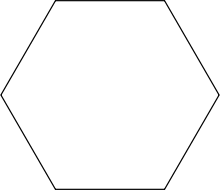The image above is a hexagon.

To compute the area of a hexagon, one essential parameter is needed and this parameter is length of side (a).

The formula for calculating the area of a hexagon:

A = (a2)3√32

Where;
A = Area of the hexagon
a = Length of side

Let’s solve an example;
Find the area of a hexagon when the length of side is 35 cm.

A = (a2)3√32
A = (352)3√32
A = 1225 x 3(1.73)2
A = 1225 x 5.1962
A = 6365.2872
A = 3182.64

Therefore, the area of the hexagon is 3182.64 cm2.

Calculating the length of side (a) using the area of the hexagon.

a = √(2A3√3)

Where;
a = length of side
A = Area of the hexagon

Let’s solve an example;
Given that the area of the hexagon is 120 cm2. Find the length of side?

This implies that;
A = Area of the hexagon = 120 cm2

a = √(2A3√3)
a = √(2 x 1205.196)
a = √(2405.196)
a = √46.189
a = 6.796

Therefore, the length of side (a) is 6.796 cm.

## How to Calculate and Solve for the Length of a Side and Perimeter of a Pentagon | Nickzom Calculator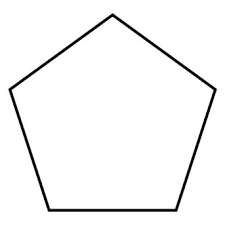The image above is a pentagon.

To compute the perimeter of a pentagon, one essential parameter is needed and this parameter is length of side (a).

The formula for calculating the perimeter of a pentagon:

P = 5a

Where;
P = Perimeter of the pentagon
a = length of side

Let’s solve an example;
Find the perimeter of a pentagon when the length of side (a) is 25 cm.

This implies that;
a = length of side = 25 cm

P = 5a
P = 5 x 25
P = 125

Therefore, the perimeter of the pentagon is 125 cm.

Calculating the length of side using the perimeter of the pentagon.

a = P / 5

Where;
a = Length of side
P = Perimeter of the pentagon

Let’s solve an example;
Given that the area of the pentagon is 250 cm. Find the length of side?

This implies that;
P = Perimeter of the pentagon = 250 cm

a = P / 5
a = 250 / 5
a = 50

Therefore, the length of side (a) is 50 cm.